# When a 200 g mass attached to a horizontal spring (k= 25 N/m) is pushed 10...

When a 200 g mass attached to a horizontal spring (k= 25 N/m) is pushed 10 cm into the spring and released, it undergoes simple harmonic motion. Find the quantities below for this oscillating system: (a) The angular frequency (rad/sec) b) Th Period of the oscilation (sec) c) The frequency (Hz) d) The maximum speed (m/s) (e) Maximum acceleration (m/s2)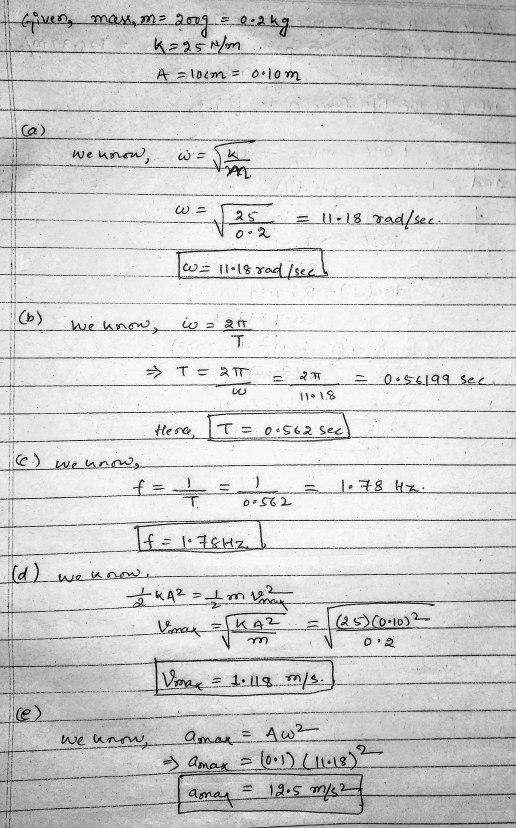#### Earn Coin

Coins can be redeemed for fabulous gifts.

Similar Homework Help Questions
• ### When a  200 g mass attached to a horizontal spring (k= 25 N/m) is pushed 10 cm...

When a  200 g mass attached to a horizontal spring (k= 25 N/m) is pushed 10 cm into the spring and released, it undergoes simple harmonic motion.  Find the quantities below for this oscillating system (a) The angular frequency (rad/sec) (b) Th Period of the oscilation (sec) (c) The frequency (Hz) (d) The maximum speed (m/s) (e) Maximum acceleration (m/s2)

• ### QUESTION 10 When a 200 g mass attached to a horizontal spring (k= 25 N/m) is...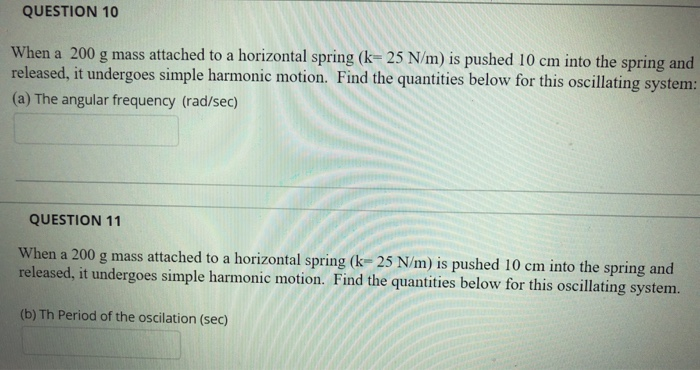QUESTION 10 When a 200 g mass attached to a horizontal spring (k= 25 N/m) is pushed 10 cm into the spring and released, it undergoes simple harmonic motion. Find the quantities below for this oscillating system: (a) The angular frequency (rad/sec) QUESTION 11 When a 200 g mass attached to a horizontal spring (k-25 N/m) is pushed 10 cm into the spring and released, it undergoes simple harmonic motion. Find the quantities below for this oscillating system. (b) Th...

• ### When a 200 g mass attached to a horizontal spring (k=25 N/m) is pushed 10 cm...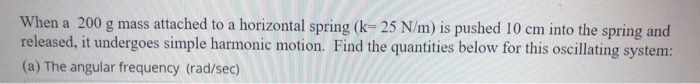When a 200 g mass attached to a horizontal spring (k=25 N/m) is pushed 10 cm into the spring and released, it undergoes simple harmonic motion. Find the quantities below for this oscillating system: (a) The angular frequency (rad/sec)

• ### When a  200 g mass attached to a horizontal spring (k= 25 N/m) is pushed 10 cm...

When a  200 g mass attached to a horizontal spring (k= 25 N/m) is pushed 10 cm into the spring and released, it undergoes simple harmonic motion.  Find the quantities below for this oscillating system: (a) The angular frequency (rad/sec)

• ### When a  200 g mass attached to a horizontal spring (k= 25 N/m) is pushed 10 cm...

When a  200 g mass attached to a horizontal spring (k= 25 N/m) is pushed 10 cm into the spring and released, it undergoes simple harmonic motion.  Find the quantities below for this oscillating system. (d) The maximum speed (m/s)

• ### A 23.3-kg mass is attached to one end of a horizontal spring, with the other...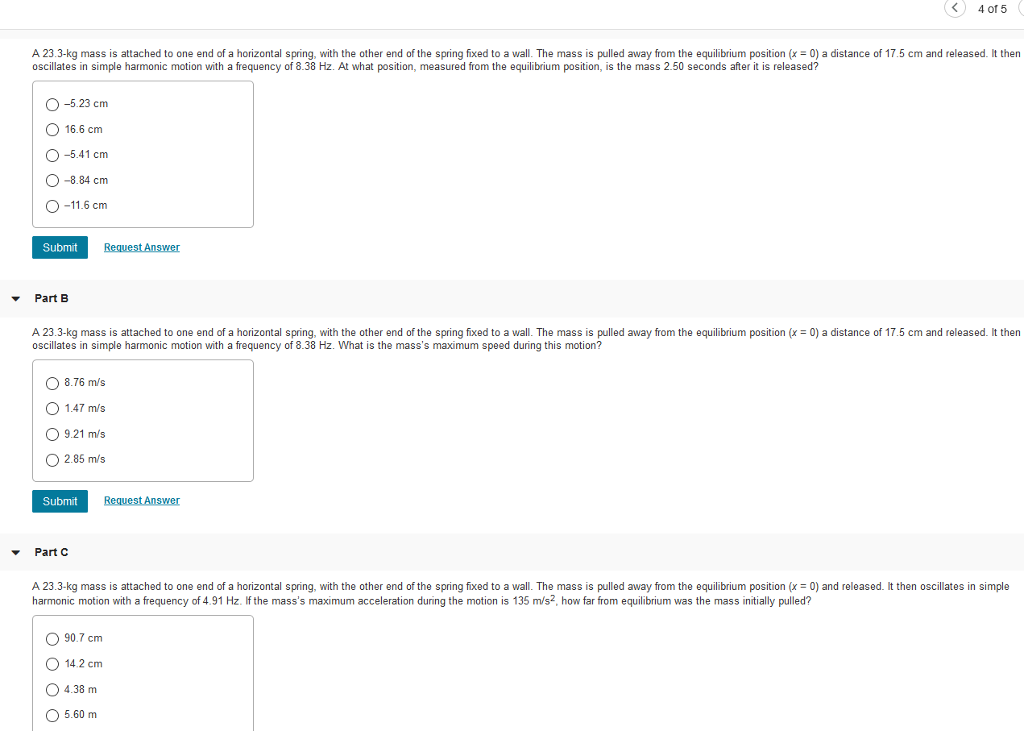A 23.3-kg mass is attached to one end of a horizontal spring, with the other end of the spring fixed to a wall. The mass is pulled away from the equilibrium position (x = 0) a distance of 17.5 cm and released. It then oscillates in simple harmonic motion with a frequency of 8.38 Hz. At what position, measured from the equilibrium position, is the mass 2.50 seconds after it is released? –5.23 cm 16.6 cm –5.41 cm –8.84...

• ### Problem3 A (2+0.1y) kg block attached to a spring undergoes simple harmonic motion described by x...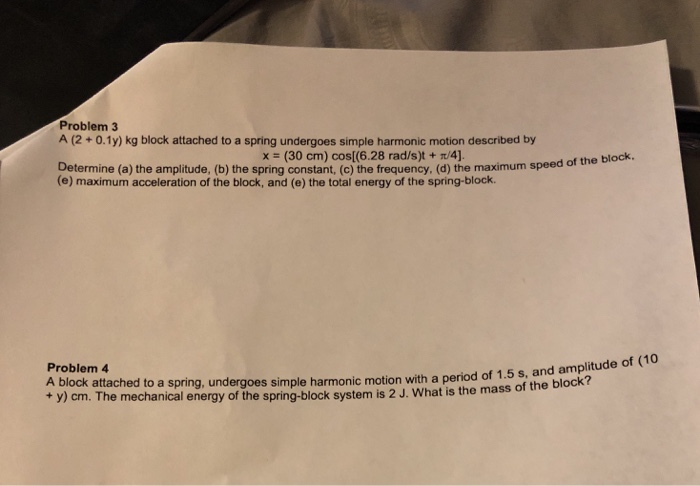Can you please answer both questions, Y=0 Problem3 A (2+0.1y) kg block attached to a spring undergoes simple harmonic motion described by x (30 cm) cos[(6.28 rad/s)t + /4) Determine (a) the amplitude, (b) the spring constant, (c) the frequency, (d) the maximum speed (e) maximum acceleration of the block, and (e) the total energy of the spring-block. of the block Problem 4 A block attached to a spring, undergoes simple harmonic motion with a period of 1.5 + y)...

• ### A 1.30-kg object is attached to a spring and placed on frictionless, horizontal surface. A horizontal...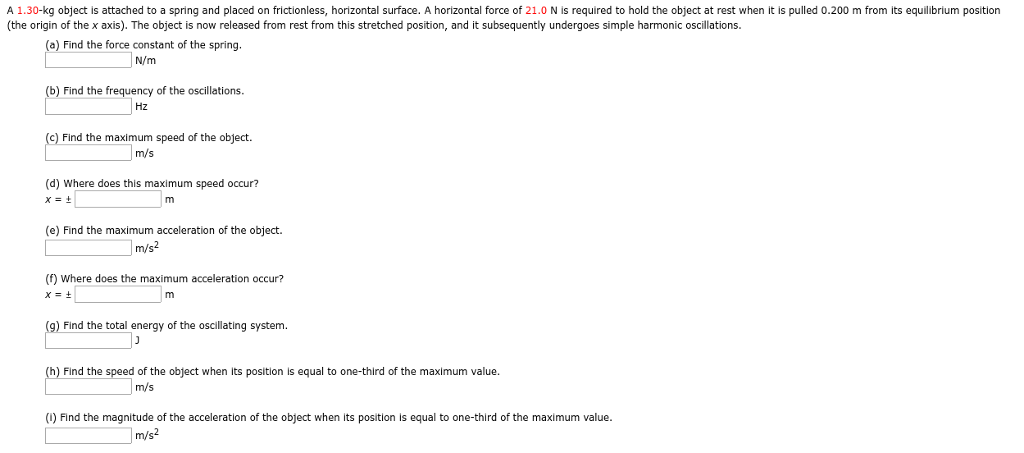A 1.30-kg object is attached to a spring and placed on frictionless, horizontal surface. A horizontal force of 21.0 N is required to hold the object at rest when it is pulled 0.200m from its equilibrium position...... Would you write out the intermediate steps, too, please? A 1.30-kg object is attached to a spring and placed on frictionless, horizontal surface. A horizontal force of 21.0 N is required to hold the object at rest when it is pulled 0.200 m...

• ### A 3.70 kg object is attached to a spring and placed on frictionless, horizontal surface. A...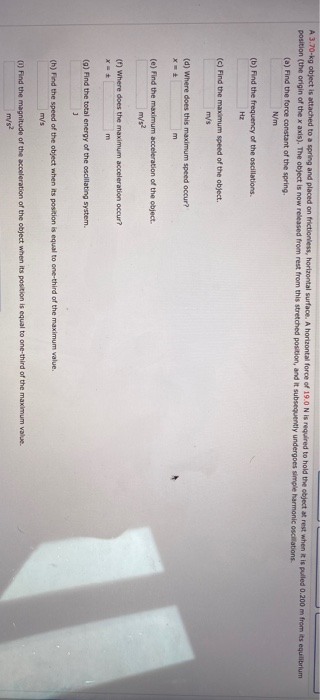A 3.70 kg object is attached to a spring and placed on frictionless, horizontal surface. A horizontal force of 19.0 N is required to hold the object at rest when it is pulled 0.200 m from its equilibrium position (the origin of the x axis). The object is now released from rest from this stretched position, and it subsequently undergoes simple harmonic oscillations (a) Find the force constant of the spring, N/m (b) Find the frequency of the oscillations Hz...

• ### 1) A 7.5kg mass attached to a spring with a spring constant of 365 N/m oscillates...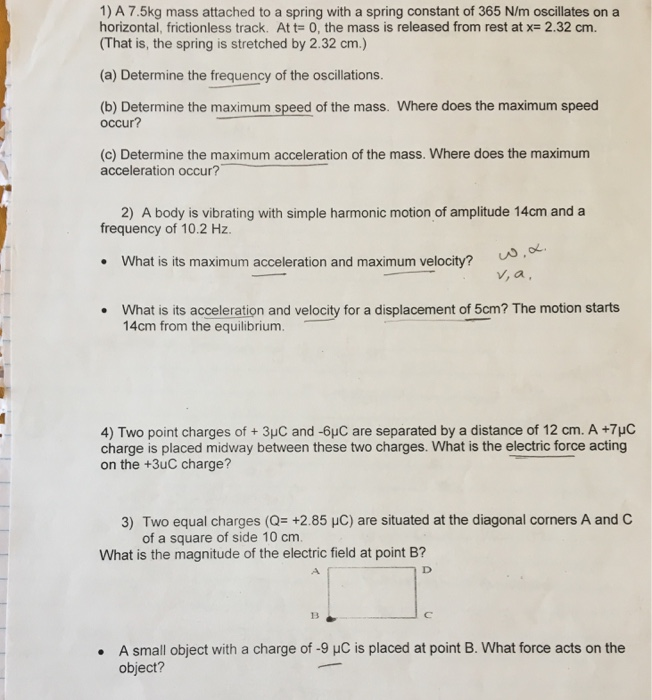1) A 7.5kg mass attached to a spring with a spring constant of 365 N/m oscillates on a horizontal, frictionless track. Att 0, the mass is released from rest at x-2.32 cm. (That is, the spring is stretched by 2.32 cm.) (a) Determine the frequency of the oscillations. (b) Determine the maximum speed of the mass. Where does the maximum speed occur? (c) Determine the maximum acceleration of the mass. Where does the maximum acceleration occur? 2) A body is...

Free Homework App